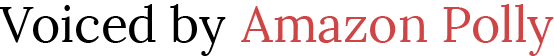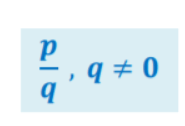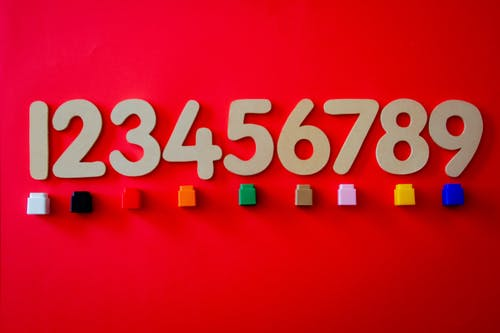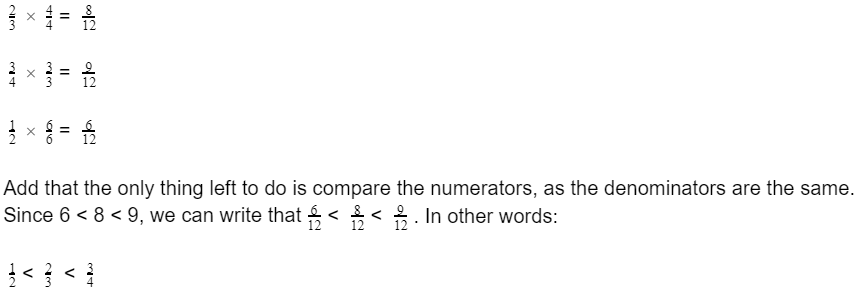# Teach Kids How to Compare and Order Rational Numbers

 Would You Rather Listen to the Lesson?In the 7th grade, students learn how to define, as well as how to compare and order rational numbers. They also learn how to understand statements of order for rational numbers that are set in real life.

Math teachers can make these lessons on rational numbers more exciting and engaging by adopting a number of teaching strategies. In this article, we bring you a few such strategies. Read on and have your students comparing and ordering rational numbers in no time!

## Teaching Strategies on How to Compare and Order Rational Numbers

### What Are Rational Numbers?

You may want to start your lesson by defining rational numbers. Explain to students that rational numbers are all the numbers that can be expressed as a ratio of two integers, in the form of a⁄b, where b does not equal 0. You can also present it in the following way on the whiteboard:Provide a few examples of rational numbers and explain to students why these are rational numbers:

• 4 is a rational number because we can express it as a ratio of two integers as 4⁄1
• 2⁄4 is a rational number because it’s expressed as a ratio of two integers
• 2.5 is a rational number because we can express it as a ratio of two integers as 2⁄5
• √16 is a rational number because we can simplify it as 4, and express 4 as a ratio of two integers as 4⁄1
• 0.5 is a rational number because we can express it as a ratio of two integers as 1⁄2### How to Compare Rational Numbers

Now that students are confident in their skills to define and identify rational numbers, you can move on to teaching how to compare them. For starters, remind students that we call the bottom number in fractions a denominator, whereas the top number is called a numerator.

For instance, in 3⁄4, the numerator is 3, and the denominator is 4. Then, explain that to compare rational numbers with the same denominator, we simply need to compare the numerators of the given numbers.

Point out that the fraction that has a greater numerator will be greater in this case, and the fraction that has a smaller numerator is smaller. Present an example on the whiteboard of two fractions with the same denominator and demonstrate this process.

Then, point out to students that sometimes we need to compare rational numbers with different denominators. To be able to do this, we first need to make the denominators of both fractions the same.

How can we make them the same? Highlight that we’ll start by finding the least common denominator (LCD). We’ll first list the multiples of the two different bottom numbers (denominators).

You can also remind students the multiples of an integer include all the numbers that we can make when we multiply that integer by another integer. We’ll stop making multiples as soon as we find an answer that’s the same for both numbers, i.e. the LCM.

This will be the new common denominator. At this point, we can proceed with multiplying the numerators and denominators of the fractions with appropriate numbers to get equivalent fractions with the identified common denominator.

Afterward, we can simply compare their numerators and determine which fraction is greater based on that. Present an example of two fractions with a different denominator and demonstrate this process.

#### Example 1:

Write the following two fractions:

3⁄4 and 1⁄4

Point out to students that since we can observe that they both have the same denominator, that is, 4, we will simply focus on their numerators. Since 3 is greater than 1, we can conclude that 3⁄4 is greater than 1⁄4.

You can also use the inequality symbols, such as greater than (>), less than (<), or equal to (=).

If we compare the two rational numbers with the mentioned symbols, we’ll get the following statement:

3⁄4 > 1⁄4

#### Example 2:

Write the following two fractions on the whiteboard:

2⁄3 and 7⁄9

Point out to students that since we can observe that the denominators are different, that is, 3 and 9, we first need to make them the same. To this end, we will find the least common multiple (LCM) of 3 and 9.

We can easily observe that this is 9, as 9 is divisible by 9 and 9 is divisible by 3 as well. Since 7⁄9 already has 9 as its denominator, we will only transform 2⁄3 to an appropriate fraction with a denominator 9.

2⁄3 × ?⁄? = ?⁄9

How to transform 23into a fraction with a denominator 9?

2⁄3 × 3⁄3= 6⁄9

Point out that we multiply 2⁄3 by 3⁄3 because, in order to reach 9 as a multiple in the denominator, we need to multiply 3 by 3.

Then, add that we had to multiply the numerator, that is, 2, by the same number by which we multiplied the denominator so that the value of the initial fraction remains unchanged.

Now that both of our fractions have the same denominator, i.e. 9, we can proceed with comparing their numerators and thus determining which fraction is greater. Since 6 is less than 7, we can conclude that 6⁄9 is less than 7⁄9, or:

6⁄9 < 7⁄9

### How to Order Rational Numbers

Once students are confident in their skills to compare rational numbers, they can move on to learning how to order them. Explain that when ordering rational numbers, we start by comparing the given numbers and then we order them from the smallest to the greatest or vice versa.

Present an example. For instance, let’s say we want to order the fractions 2⁄3, 3⁄4, 1⁄2 from least to greatest. We first need to make the denominators of all three fractions the same. By applying the LCM method explained above, we can identify 12 as the least common denominator.

Then, we’ll transform each of the three fractions to an equivalent fraction with 12 as denominator:If you have the technical means in your classroom, you can also incorporate multimedia materials in your lesson on how to compare and order rational numbers. For instance, you can use videos after you’ve reviewed rational numbers.

This video contains simple guidelines on how to compare rational numbers and then order them from least to greatest, whereas this video explains how to find LCD or the least common denominator.

## Activities to Practice Comparing and Ordering Rational Numbers

### Comparing Rational Numbers Jeopardy Game

This is an online game where students can practice comparing rational numbers. To use this game in your classroom, make sure there is a suitable number of devices and a decent internet connection.

Pair students up and provide instructions. Students play together on the same device and choose the option of two separate avatars. They click on their avatar before answering a question.

Each player is presented with questions where they are asked to compare the given rational numbers. If they answer correctly, they can score from 100 to 400 points, depending on the difficulty of the question.

In the end, the two players compare their scores. The student with the biggest score wins the game. Homeschooling parents can also use this game with their children by choosing the ‘single avatar’ option.

### Get Them in Order! Game

This is a game that will help students improve their ability to quickly understand statements of order for rational numbers. To implement this game in your class, you’ll need a timer and several sets of task cards.

You can create the cards from construction paper on your own. Each task card contains one rational number. Create a few sets (depending on the number of students in your class). Each set has around 10 cards.

Divide students into groups of 3 or 4 and hand out one set of cards per group. Provide instructions for the game. Explain to students that they have to arrange the rational numbers on the cards from the smallest to the greatest.

Provide a few minutes for this and set a timer. When the time is up, each group shares their work and explains what rules and strategies they used in order to arrange the numbers in ascending order.

## Before You Leave…

If you enjoyed these strategies and activities on teaching kids how to compare and order rational numbers, and you’re looking for more math materials for children of all ages, sign up for our emails and get loads of free lessons and content!

Make sure to also head over to our blog – you’ll find plenty of awesome resources for your class!